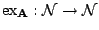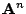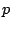# Constraint satisfaction problems and expressiveness

Victor Dalmau

Universitat Pompeu Fabra

Constraint satisfaction problems arise in a wide variety of domains, such as combinatorics, logic, and artificial intelligence. In recent years, much effort has been directed towards classifying the complexity of all families of constraint satisfaction problems. It has been shown that it is possible to associate to every subclass of the CSP an algebrasuch that the complexity the corresponding CSP is completely determined by the properties of.

Letbe any algebra over a finite universe. We define the expressiveness ofas the mappingthat given a natural numberreturns the number subuniverses of. Some algebrasthat lead to tractable cases of the CSP such as Mal'tsev algebras, or algebras containing a near-unanimity operations among their term operations, satisfy the following property:, for some polynomial. We call any such algebra polynomially expressible. We conjecture that all polynomially expressible algebras give rise to families of constraint satisfaction problems solvable in polynomial time. In this talk we shall present some initial results in this direction.

2005-05-23